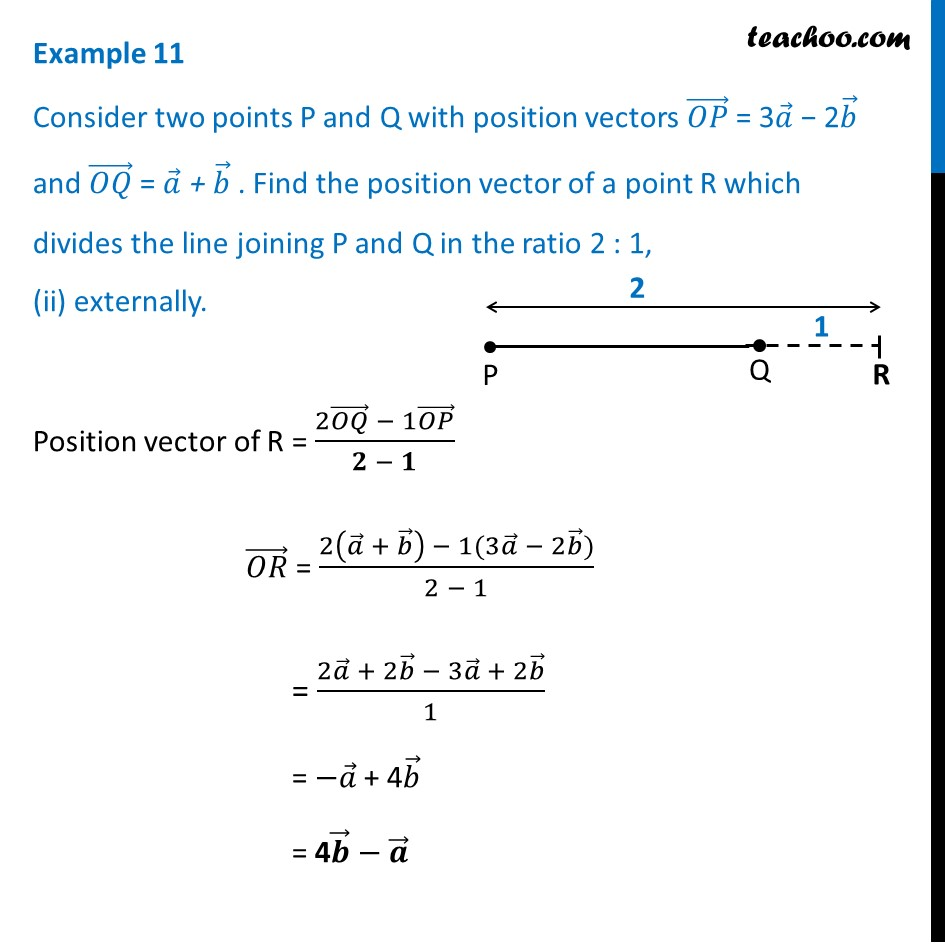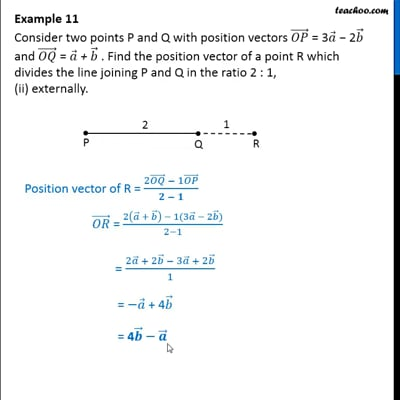Examples

Chapter 10 Class 12 Vector Algebra
Serial order wiseThis video is only available for Teachoo black users

Maths Crash Course - Live lectures + all videos + Real time Doubt solving!

### Transcript

Example 11 Consider two points P and Q with position vectors (𝑂𝑃) ⃗ = 3𝑎 ⃗ − 2𝑏 ⃗ and (𝑂𝑄) ⃗ = 𝑎 ⃗ + 𝑏 ⃗ . Find the position vector of a point R which divides the line joining P and Q in the ratio 2 : 1, (ii) externally. Position vector of R = (2(𝑂𝑄) ⃗ − 1(𝑂𝑃) ⃗)/(𝟐 − 𝟏) (𝑂𝑅) ⃗ = (2(𝑎 ⃗ + 𝑏 ⃗ ) − 1(3𝑎 ⃗ − 2𝑏 ⃗))/(2 − 1) = (2𝑎 ⃗ + 2𝑏 ⃗ − 3𝑎 ⃗ + 2𝑏 ⃗)/1 = −𝑎 ⃗ + 4𝑏 ⃗ = 4𝒃 ⃗ − 𝒂 ⃗